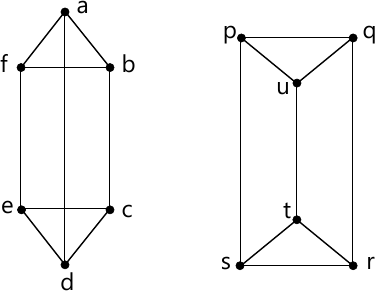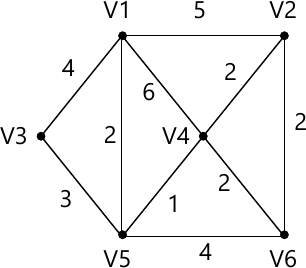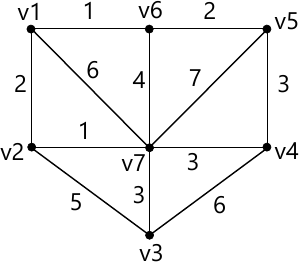MORE IN Discrete Structures
SPPU Information Technology (Semester 3)
Discrete Structures
May 2014
Total marks: --
Total time: --
INSTRUCTIONS
(1) Assume appropriate data and state your reasons
(2) Marks are given to the right of every question
(3) Draw neat diagrams wherever necessary

Answer any one question from Q1 and Q2
1 (a) Explain the principle of inclusion and exclusion.
Assume that 100 out of 120 students study at least one of the three languages Japanese, French and Russian. It is given that 65 students study Japanese, 45 study French and 42 study Russian. 20 students study Japanese and French, 25 students study Japanese and Russian and 15 study French and Russian. Find the number of students who study:
i) Only Japanese and French but not Russian
ii) Only Japanese and Russian but not French.
6 M
1 (b) Find a recurrence relation to calculate the number of bit strings of length 'n' that contains a pair of consecutive zeros. Using the same recurrence relation find the number of strings of length 7 containing 2 consecutive zeros.
6 M

2 (a) Prove by mathematical induction for n≥1. [ 1 \times 2 + 2 \times 3 + \cdots + n (n+1) = \dfrac {n (n+1)(n+2)}{3} .]
6 M
2 (b) Let R = {(a, b), (b, a), (b, c), (c, d), (d, a)} over the set A= {a, b, c, d}. Find transitive closure using Warshall's algorithm.
6 M

Answer any one question from Q3 and Q4
3 (a) Define and illustrate with example: i) Ring
ii) Integral Domain
iii) Fields
6 M
3 (b) Determine whether the graphs are isomorphic:6 M

4 (a) Define and illustrate with examples
i) Monoid
Semi-group
Group
6 M
4 (b) Solve the following travelling salesman problem using nearest neighbourhood method.
 a b c d e a 0 58 98 147 135 b 58 0 142 167 133 c 98 142 0 113 137 d 147 167 113 0 56 e 135 133 137 56 0
6 M

Answer any one question from Q5 and Q6
5 (a) Find the MST for the graph given below using Kruskal's algorithm.6 M
5 (b) Use Huffman coding to encode the following symbols.
7 M

6 (a) Find the MST for the graph given below using Prism's algorithm.6 M
6 (b) Represent the following expression in:
i) Using a binary tree
ii) Post-fix notation
iii) Pre-fix notation
((x+2) ↑ 3)×(y?(3+x)) ? 5
7 M

Answer any one question from Q7 and Q8
7 (a) A coin was chosen at random and tossed. The probability that a fair coin was chosen and 'head' as an outcome is 1/3. The probability that a fair coin was chosen and 'tail' as an outcome is also 1/3. The probability that an unfair coin was chosen and 'head' as an outcome is 1/12. The probability that an unfair coin was chosen and 'tail' as an outcome is 1/4. Find the conditional probability that
i) Unfair coin chosen given that 'head' is the outcome.
ii) 'Head' is the outcome given that unfair coin is chosen.
7 M
7 (b) How many ways are there to handover 5 cards to each of 4 players from standard deck of 52 cards.
6 M

8 (a) State and explain Baye's theorem.
7 M
8 (b) How many different strings can be made by reordering the letters of the word SUCCESS?
6 M

More question papers from Discrete Structures